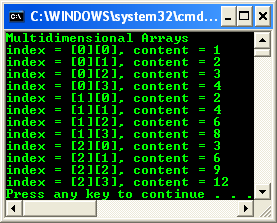4. # Multidimensional Arrays

Passing Arrays to Functions

Passing arrays to functions introduces one complication because of the fact that an array has no knowledge of its size or contents. As you’ll see shortly, when you pass an array to a function, you pass only the starting address, which means that you have to figure out some way of passing the size information along with the array when you call the function. Normally this is accomplished in one of two ways:

1. Pass the size as an explicit parameter to the function call.

2. Make sure that the array is always terminated by a unique marker value so that the function can tell when the end of the data has been reached.

How Do Native Arrays Work?

A native array in C++ isn’t an object; it’s simply a collection of values strung together in memory. So a 10-element array of integers consists of 10 integers one after the other in memory. The name of the array is a pointer to the first element, so when you declare an array like this:

int foo;

you’re telling the compiler to reserve memory large enough to hold 10 integers and return you the address as foo. When you access an array element, you’re actually specifying the offset from this address, so that foo means “offset one int from the address foo, and use what is stored there.” This explains why array indexing starts from 0: an index of 0 denotes an offset of zero from the start address, so it means the first element.

Once the compiler has allocated the space, pretty much all it knows about an array is its starting address. When you provide an offset in terms of an array index, the compiler generates code to access that piece of memory. And if you’ve got it wrong and stepped outside the bounds of the array, you can end up reading or writing somewhere inappropriately. In fact, deliberately accessing outside the bounds of arrays has been the basis for many security attacks on programs and systems over the years, for example buffer overflow attack and also bugs. To finish this brief explanation, note that there’s a close link between arrays and pointers, so close, in fact, that any array access can be written using pointer notation instead of array notation, as shown here:

// These two are equivalent

n = arr;

n = *(arr + 3);

In the second example, the compiler is being told to dereference the location in memory whose distance from address arr is the size of three int variables.

1.        Let’s investigate passing an array to a function. Continue with the project from the previous exercise; reopen it if necessary. Add the following function definition immediately after the using namespace System; line:

void func(int arr[], size_t size)

{

for(size_t i=0; i<size; i++)

{

arr[i] = i+2;

Console::WriteLine("index = {0}, content = {1}", i, arr[i]);

}

}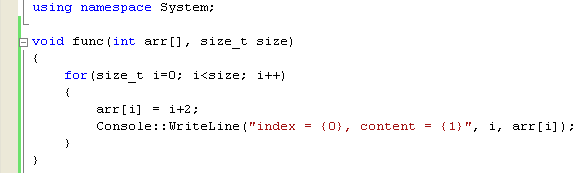The first argument to the function tells the compiler that the address of an array is going to be passed, which is equivalent to passing a pointer. It’s very common to see int* used instead. The second argument passes the size of the array, in effect, the amount of memory pointed to by the first argument. The size_t type is a typedef for unsigned int, and it’s good practice to use this type for integer arguments that denote sizes, lengths, or dimensions. The function prints out the array by using the size, just as before.

2.        Call the function from the main() routine like this:

func(arr, 10);

3.        So our new code is shown below. Build and run your project.

// Trad.cpp : main project file.

#include "stdafx.h"

using namespace System;

void func(int arr[], size_t size)

{

for(size_t i=0; i<size; i++)

{

arr[i] = i+2;

Console::WriteLine("index = {0}, content = {1}", i, arr[i]);

}

}

int main(array<System::String ^> ^args)

{

// Create an array

int arr;

func(arr, 10);

return 0;

}

Output: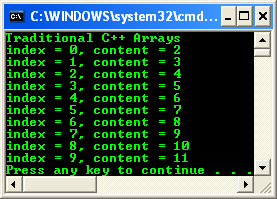What if the array size was changed at some point? You can make your code more robust by calculating the number of elements in the array automatically using the sizeof operator, like this:

func(arr, sizeof(arr)/sizeof(arr));

The sizeof operator returns the size of its argument in bytes, where the argument can be a variable name or a type name. Using sizeof on an array returns the total size of the array in bytes, in this case, 40 bytes. When divided by the size of one element, 4 bytes, you’re left with the number of elements in the array.

Initializing Arrays

It’s possible to initialize arrays at the point of declaration, as shown in the following syntax fragment:

`int arr = { 1, 2, 3, 4 };`

The values to be used for initialization are provided as a comma-separated list in braces ({}) on the right-hand side of an assignment; these values are known as an aggregate initializer. The compiler is clever enough to figure out how many values are in the list, and it will dimension the array to fit if you don’t provide a value.

// Dimension the array automatically

int arr[] = { 1, 2, 3, 4 };

If you give a dimension and then provide too many values, you’ll get a compiler error. If you provide too few values, the initial values you give will be used to initialize the array starting from element zero, and the remaining elements will be set to zero.

Multidimensional Arrays

Multidimensional arrays in C++ are an extension of the single-dimensional variety, in that a two-dimensional array is actually an array of single-dimensional arrays. So in C++, arrays of higher dimensions are all built out of single-dimensional arrays. The following short exercise shows how to create and use a two- dimensional array.

1.        Open Visual C++/Studio, and create a new CLR Console Application project named MultiD.2.        Open the source file MultiD.cpp and add the following code to the main() function:

int main(array<System::String ^> ^args)

{

Console::WriteLine(L"Multidimensional Arrays");

// Create a 2D array

int arr;

// Fill the array

// the row...

for(int i=0; i<4; i++)

// the column

for(int j=0; j<5; j++)

arr[i][j] = (i+1)*(j+1);

return 0;

}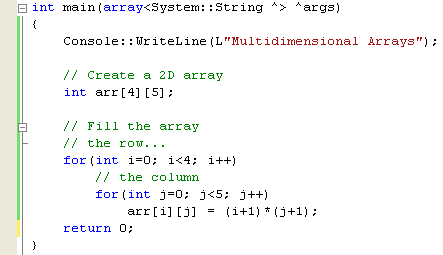Note that a two-dimensional array is declared by using two sets of square brackets. You don’t put the two values inside one set of brackets, as you do in many other languages, and for higher order arrays, you simply add more sets of square brackets. As with single-dimensional arrays, you have to give the size at compile time, and the indices of each dimension vary from zero to one less than the declared size. Array elements are also accessed using two sets of square brackets.

3.        Print out the array using an extension of the method for printing out the elements of the single-dimensional array, as follows:

// Print the array content

for(int i=0; i<4; i++)

{

for(int j=0; j<5; j++)

Console::Write("{0} ", (Object^)arr[i][j]);

Console::WriteLine();

}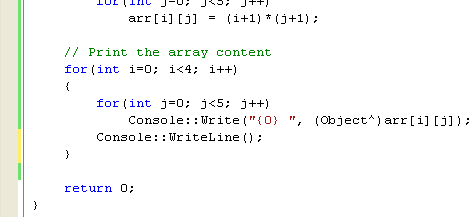4.        Build and run your project. The following is the expected output.Notice that one row of the array gets printed on one line. The inner loop prints a single row using repeated calls to Console::Write. To format the output, the array element has to be boxed using a call to the Object^ keyword (old version C++ .NET uses __box) . After each row has been output, a call to Console::WriteLine outputs a new line.

5.   To pass a multidimensional array to a function, use two empty sets of square brackets (for example, int arr[][]) and specify the dimension information as before. More meaningful version is shown below.

// MultiD.cpp : main project file.

#include "stdafx.h"

using namespace System;

int main(array<System::String ^> ^args)

{

Console::WriteLine(L"Multidimensional Arrays");

// Create a 2D array

int arr;

// Fill the array

// the row...

for(int i=0; i<3; i++)

// the column

for(int j=0; j<4; j++)

arr[i][j] = (i+1)*(j+1);

// Print the array index & content

for(int i=0; i<3; i++)

{

for(int j=0; j<4; j++)

Console::WriteLine("index = [{0}][{1}], content = {2}", i, j, arr[i][j]);

}

return 0;

}

Output: